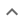•# Raising to a power

We think you wrote:

###This solution deals with raising to a power.

Solution found(a^(-8)b^(-6)*2^2)/(1*3^2)

## Step by Step Solution### Reformatting the input :

(1): "^-2" was replaced by "^(-2)".

## Step  1  :

            b2
Simplify   ——
2


#### Equation at the end of step  1  :

              b2
((((3•(a3))•——)•a)•b)-2
2
Step  2  :

#### Equation at the end of step  2  :

           b2
(((3a3 • ——) • a) • b)-2
2


## Step  3  :

#### Equation at the end of step  3  :

    3a3b2
((————— • a) • b)-2
2
Step  4  : 4.1    a4 raised to the minus 2 nd power = a( 4 * -2 ) = a-8 4.2    b3 raised to the minus 2 nd power = b( 3 * -2 ) = b-6

## Final result :

  a(-8)b(-6) • 22
———————————————
1 • 32